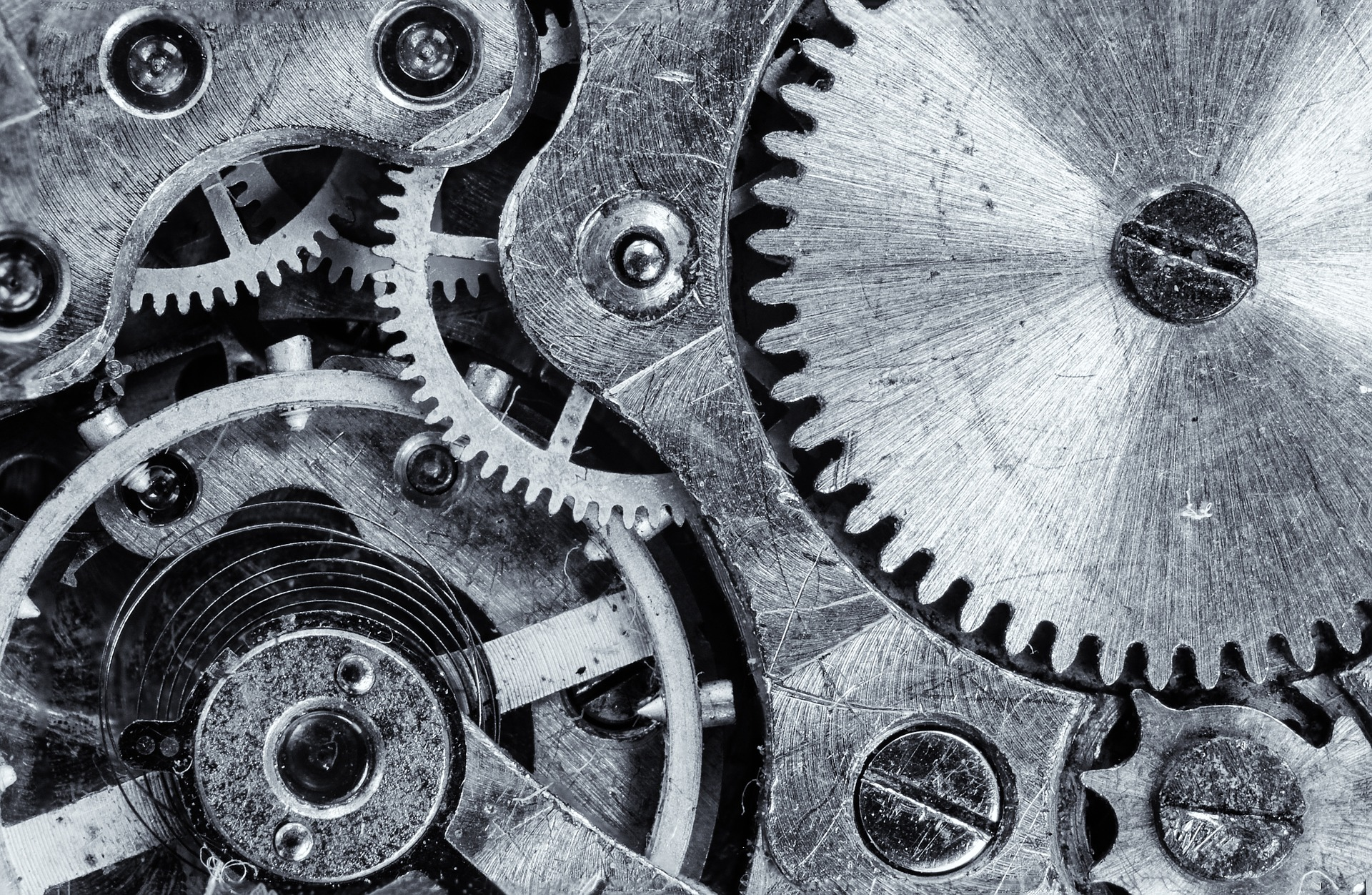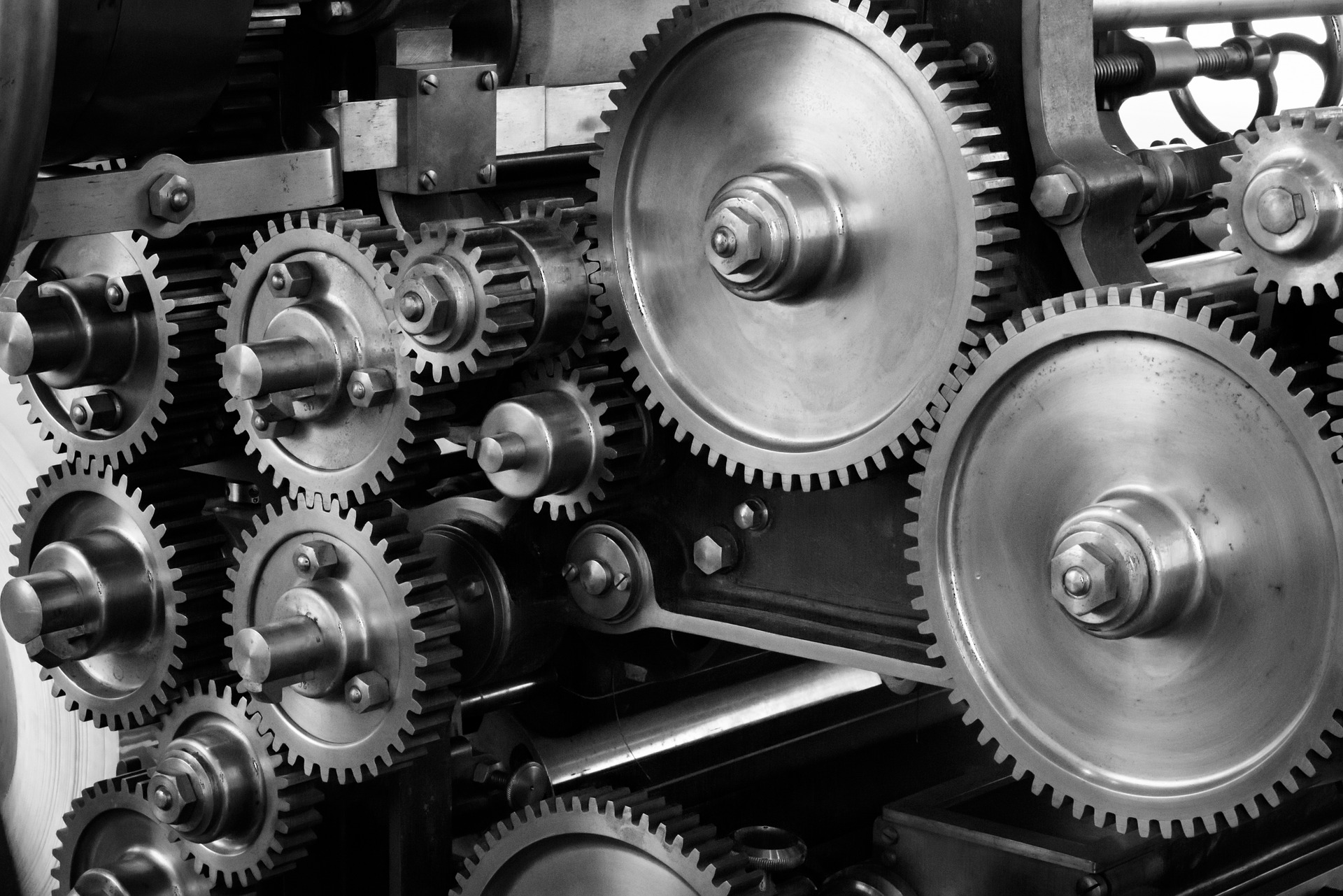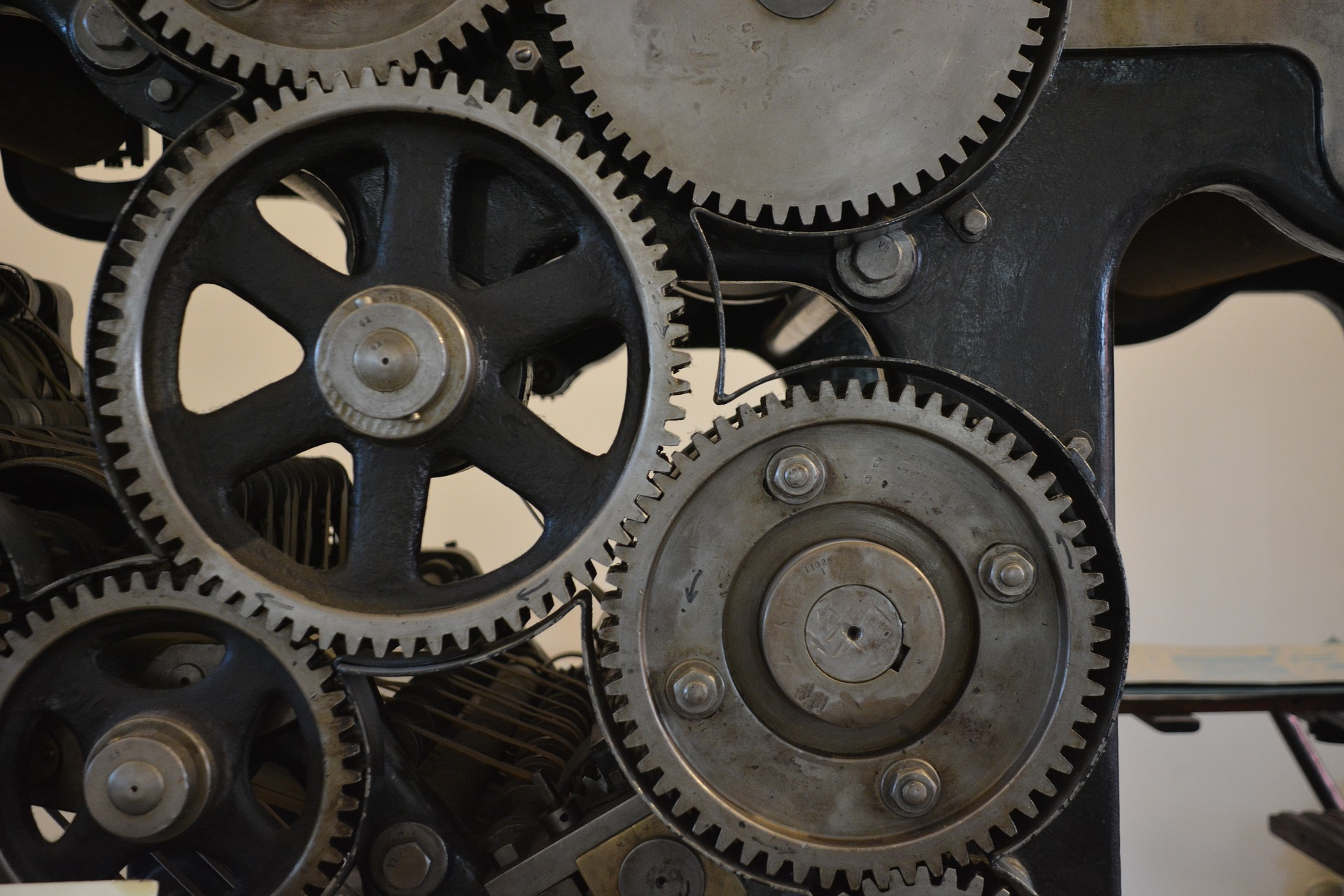## The Importance of Determining Number of Stages in Reciprocating Compressor and Bonus Calculation Spreadsheet

Three previous posts show comparison of number of stages in determining reciprocating compressor power.

Estimate reciprocating compressor power (2-stage compression)

Estimate reciprocating compressor power (3-stage compression)

Estimate reciprocating compressor power (2-stage compression)

Let’s summarize the results. Read More## Reciprocating Compressor Power Calculation Part 3 (Four-Stage Compression)

In previous post, I shared how to calculate reciprocating compressor power if number of compression stage is two and three, respectively. In this post, I will use the same problem/example to estimate reciprocating compressor power if number of compression stage is 4. In the end of this posting series, I will show you the difference of each stage and to see how important to determine number of compression stages.

The method used to calculate reciprocating compressor is the same, whether it is two-stage, three-stage, or four-stage. There are several steps which is repetitive.

# Example

Compress 2 MMscfd of gas measured at 14.65 psia and 60oF. Intake pressure is 100 psia, and intake temperature is 100oF. Discharge pressure is 900 psia. The gas has a specific gravity of 0.80 (23 MW). What is the required horse power?

Assuming number of stages is 4 Read More## Reciprocating Compressor Power Calculation Part 2 (Three-Stage Compression)

In previous post, I shared how to calculate reciprocating compressor power if number of compression stage is two. In this post, I will use the same problem/example to estimate reciprocating compressor power if number of compression stage is 3. In the end of this posting series, I will show you the difference of each stage and to see how important to determine number of compression stages.

The method used to calculate reciprocating compressor is the same, whether it is two-stage, three-stage, or four-stage. There are several steps which is repetitive. Read More## Reciprocating Compressor Power Calculation Part 1 (Two-Stage Compression)

Many of readers in of this technical blog asked me how to calculate reciprocating compressor power. Long time ago I wrote how to estimate centrifugal compressor power.

In this post I want to share how to calculate reciprocating compressor power. This calculation is used for estimation only. For more accurate results, you should consult a compressor manufacturer.

You will find that the method used in this calculation rely on graphical methods. So, please be patient in using this method.

I used calculation method as stated in GPSA.

Let’s start using example. I used example as used in GPSA. Read More